Function Repository Resource:

# TridiagonalInverse

Evaluate the inverse of a tridiagonal matrix

Contributed by: Jan Mangaldan
 ResourceFunction["TridiagonalInverse"][a,b,c] gives the inverse of the tridiagonal matrix with subdiagonal a, diagonal b and superdiagonal c.

## Examples

### Basic Examples (2)

The inverse of a 3×3 tridiagonal matrix:

 In:=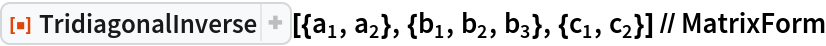Out=Multiply with the original tridiagonal matrix:

 In:=Out=### Scope (1)

TridiagonalInverse can be used with numerical entries:

 In:=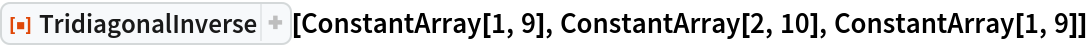Out=In:=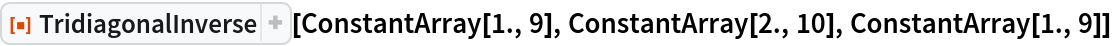Out=### Applications (2)

Construct a natural cubic spline interpolant to data:

 In:=Out=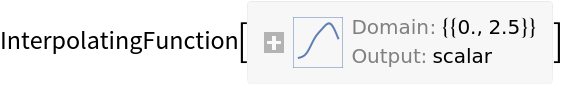Plot the interpolant along with the data:

 In:=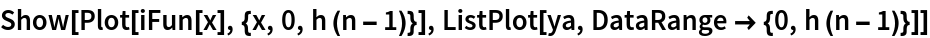Out=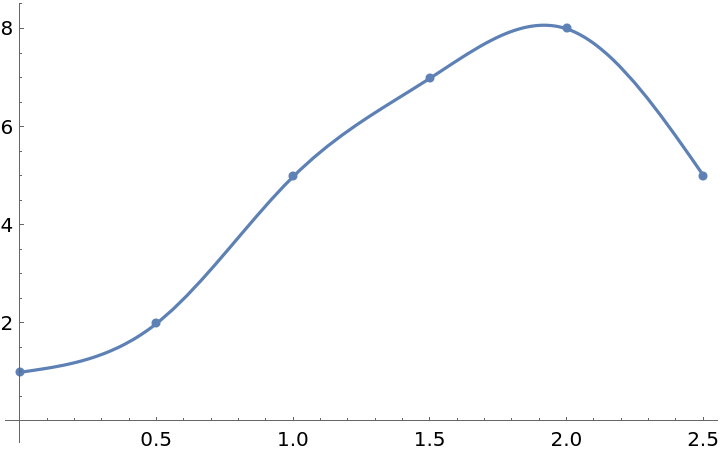Approximately solve the boundary value problem u{XMLElement[span, {class -> stylebox}, {x, XMLElement[i, {class -> ti}, {x}]}]}+u=f;u(0)=u(1)=0:

 In:=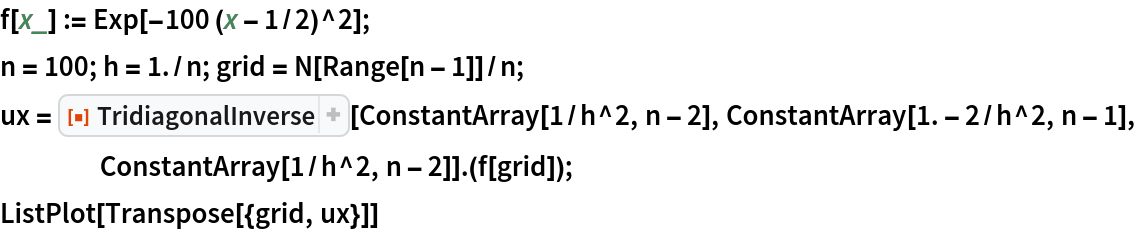Out=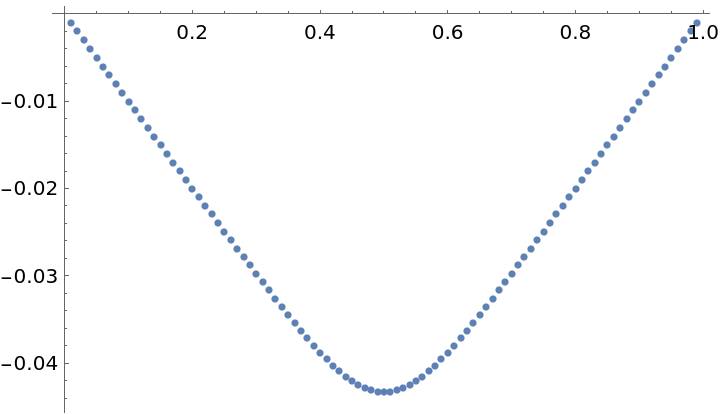Show the error compared with the exact solution:

 In:=Out=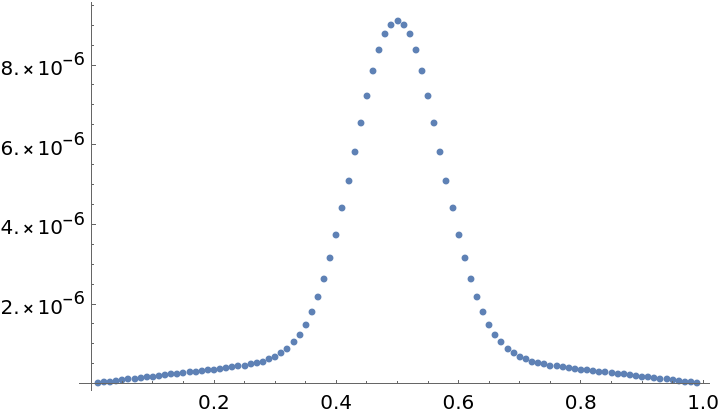### Properties and Relations (1)

TridiagonalInverse can be faster than using Inverse on a tridiagonal matrix constructed with SparseArray and Band:

 In:=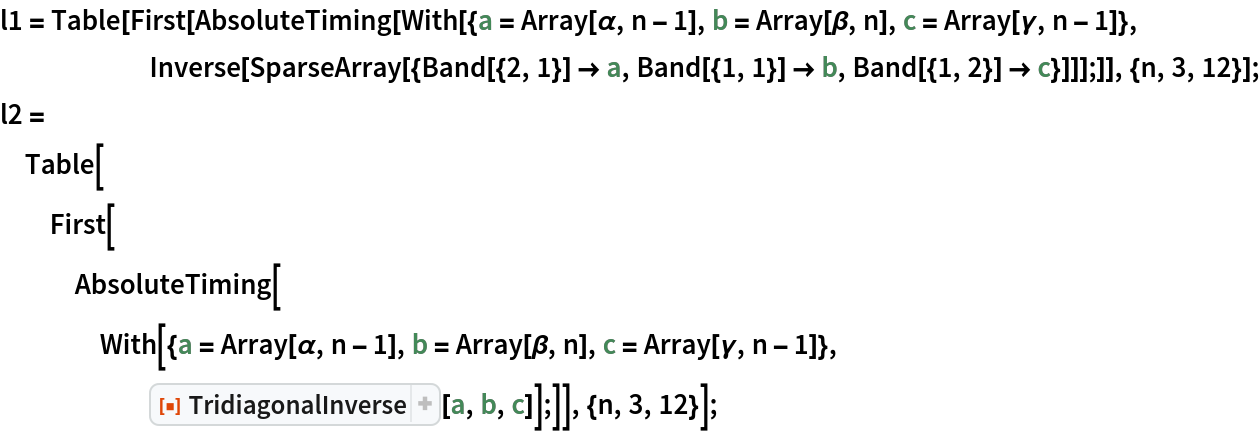In:=Out=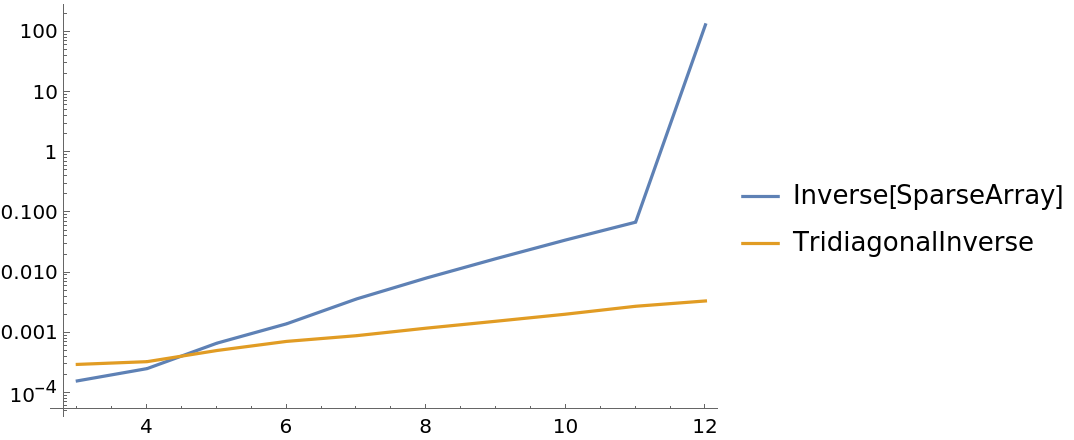## Version History

• 1.0.0 – 21 June 2021